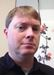# PRINCIPLES OF ECONOMICS FOR GRADES 10 - 12

Sale ends in
Teacher: JOSEPH
Customers Who Have Viewed This Course: 1479
\$199.00
\$95.00

#### 0 Lesson 1: Introduction to Economics 23:36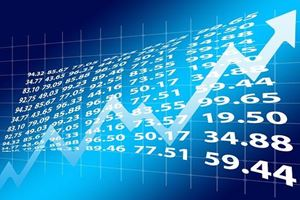Learning Objectives 1. To explain the nature of Economics 2. To explain the use of the social scientific method in Economics

#### 1 Practice Exercise 1 Solutions 05:13

Solutions to Practice Exercise 1

#### 2 Lesson 2: Microeconomics Versus Macroeconomics 12:03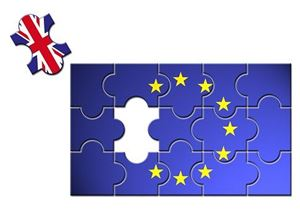This lesson distinguishes between the two main branches of the subject and explores the main categories of each branch.

#### 3 Practice Exercise 2 Solutions 03:13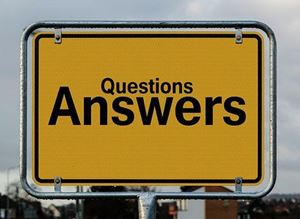Short video lesson providing answers to the practice exercise

#### 4 Lesson 3: Model-building in Economics 21:13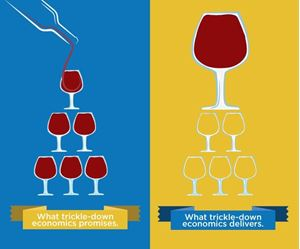This lesson describes how Economics as a social science, uses models to describe how the real world works.

#### 5 Practice Exercise 3 Solutions 03:19

Solutions to Practice exercise 3

#### 6 Lesson 4: Positive Versus Normative Economics 21:19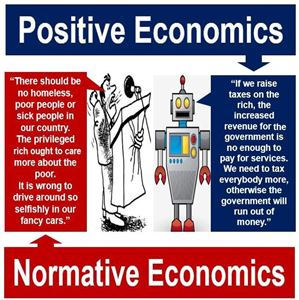This lesson examines the role of factual statements and those based on opinions in economic decision-making.

#### 7 Practice Exercise 4 Solutions 03:50

Solutions to Practice exercise 4

#### 8 Lesson 5: Basic Organizations and Economic Institutions 16:49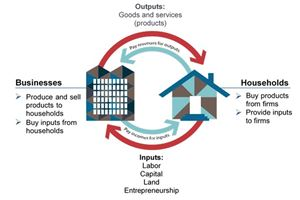Learning Objectives 1. To use the circular flow of income model to identify economic institutions 2. To explain basic economic agents and organisations.

#### 9 Practice Exercise 5 Solutions 05:22

Solutions to Practice exercise 5

#### 10 Lesson 6: The Basic Economic Problem 22:32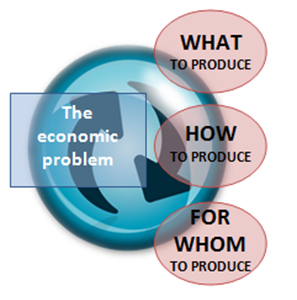The issue of scarce economic resources and how they are allocated amongst numerous competing human needs and wants is explained in this lesson.

#### 11 Practice Exercise 6 Solutions 01:47

This video lesson contains guidelines on how to respond to questions from the practice exercise for lesson 6.

#### 12 Lesson 7: Factor Markets 23:42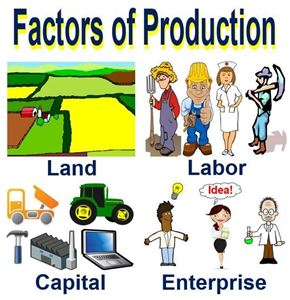Households provide inputs or factors of production that firms engage in the production of goods and services. This lesson analyses the four factors of production, how they are combined and transformed into output. The rewards that each factor receives are also explained.

#### 13 Practice Exercise 7 Solutions 02:25

Solutions to Practice Exercise 7

#### 14 Lesson 8: Product markets 18:08Product markets involve the exchange of goods between firms (producers or sellers) and households (consumers or buyers). This lesson analyses the types of goods/ services that are exchanged in the product market.

#### 15 Practice Exercise 8 Solutions 02:24

Solutions to Practice exercise 3

#### 16 Lesson 9: The Production Possibilities Curve 22:28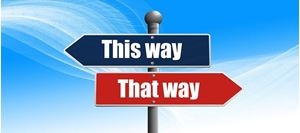Having discussed scarce resources and the unlimited human needs and wants, in the last couple of lessons, we will now consider how choices are made and the concept of opportunity cost. The production possibilities curve is used to illustrate opportunity cost.

#### 17 Practice Exercise 9 solutions 03:44

Solutions to Practice exercise 9

#### 18 Lesson 10: Key Themes in Economics 22:00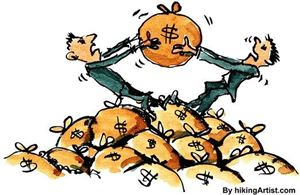This lesson examines four major themes that govern the study of Economics. Points of disagreement between economists on these themes are also discussed.

#### 19 Practice Exercise 10 Solutions 04:21

Solutions to Practice Exercise 10

#### 20 Lesson 11: What Questions Do Economic Systems Seek to Answer? 20:43In every society, there are three basic questions that have to be answered in allocating scarce resources and distributing the output produced. This lesson will discuss these questions and why they are important.

#### 21 Practice Exercise 11 Solutions 04:22

Solutions to Practice exercise 11

#### 22 Lesson 12: Economic Systems and Decision-Making 24:08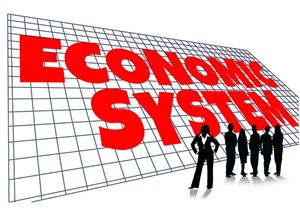This lesson outlines the basic economic decision-making units in a society and outlines examples of economic decisions that they have to make. It also identifies that factors that determine the making of rational economic decisions.

#### 23 Practice Exercise 12 Solutions 04:24

Solutions to Practice exercise 12

#### 24 Lesson 13: Free Market Economic System 34:34This lesson examines the first of the three economic systems employed by societies and countries to make decisions on how scarce resources will be allocated.

#### 25 Practice Exercise 13 Solutions 03:50

Solutions to Practice exercise 13

#### 26 Lesson 14: Planned and Mixed Economic Systems 30:32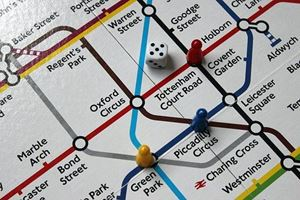We continue with our analysis of economic systems by discussing planned economies and mixed economic systems. A comparison of the three economic systems in terms of features, advantages and disadvantages wraps up the lesson as well as Section 1.

#### 27 Practice Exercise 14 04:51

Solutions to Practice Exercise 14

#### 28 Lesson 15: Competitive Markets and Demand 26:23This lesson identifies different types of markets based on what is traded and the geographical area covered. It also looks at the behaviour of buyers as illustrated by the demand curve and the law of diminishing marginal utility.

#### 29 Practice Exercise 15 Solutions 03:09

Solutions to Practice exercise 15

#### 30 Lesson 16: Movements Along The Demand Curve 20:36The use of a demand schedule (table) to plot a demand curve is illustrated. The reasons for the downward shape of the demand curve are explored. Changes in the price of a product and the impact these have on quantity demanded are also analysed.

#### 31 Practice Exercise 16 Solutions 02:09

Solutions to Practice exercise 16

#### 32 Lesson 17: The Determinants of Demand 28:26In this lesson, the non-price factors that contribute to an increase or decrease in demand (shift of the demand curve) are explained. The demand diagram is used to analyse the impact of the shifts in demand.

#### 33 Practice Exercise 17 Solutions 06:17

This video contains a guideline on how to respond to the practice exercise for lesson 17.

#### 34 Lesson 18: Elasticity of Demand 30:13The law of demand explains the direction of change in quantity demanded when the price of a product changes. However, it does not explain the magnitude of this change. this lesson explains how the concept of price elasticity of demand is used to measure the responsiveness of demand to changes in the price of the product.

#### 35 Practice Exercise 18 Solutions 06:35

Solutions to Practice Exercise 18

#### 36 Lesson 19: The Supply Curve and its Determinants 23:57This lesson starts with a definition of supply and explains how a supply schedule is used to derive a supply curve. The law of supply and movements along the same supply curve are explored. Changes in the price of a product will contribute to an increase in the quantity supplied (contraction in demand), as producers/ sellers have an incentive to produce more. However, changes in other factors that affect supply will contribute to a shift in demand. This lesson will examine the factors that affect supply and show how the supply curve shifts.

#### 37 Practice Exercise 19 Solutions 07:32

This video contains a guideline on how to respond to the practice exercise for lesson 19.

#### 38 Lesson 20: Price Elasticity of Supply (PES) 33:52According to the law of supply, there's a positive relationship between the price of a product and quantity supplied. This means a change in price causes the quantity supplied to increase. However, the law of supply does not explain the magnitude of this change. Price Elasticity of Supply helps to measure the responsiveness of quantity supplied to the change in the price of the product. This lesson explores the concept of PES and its determinants.

#### 39 Practice Exercise 20 Solutions 03:48

Solutions to Practice exercise 20

#### 40 Lesson 21: Price- Reaching an Equilibrium 25:53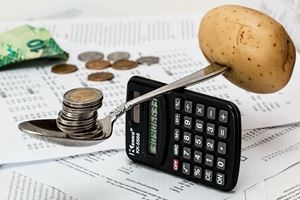Having considered the concepts of demand and supply, we now examine how the two concepts interact in the market. The point at which the two intersect, equilibrium, indicates that both consumers and producers are happy to pay the equilibrium price. This lesson analyses how markets reach and maintain the equilibrium point.At prices above or below the equilibrium price, the quantity demanded and supplied will be unequal creating a state of instability in the market. This lesson uses examples to discuss how shortages and surpluses are created in the market and how the market reacts to re-establish equilibrium.

#### 41 Practice Exercise 21 solutions 06:37

This video contains a guideline on how to respond to the practice exercise for lesson 17.

#### 42 Lesson 22: Linear Demand and Supply Functions 30:40Having examined how prices are determined in free markets, we now use a numerical analysis to explain how demand and supply curves are derived from linear demand and supply functions. In this lesson, we conduct simple equations through which we are able to see how equilibrium price and quantity are determined. We also use an example of indirect taxes to see how prices changes in the determinants of demand and supply affect the equilibrium position.

#### 43 Practice Exercise 22 Solutions 04:44

Solutions to Practice Exercise 22

#### 44 Lesson 23: Efficiency in The Market 28:38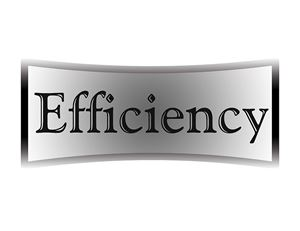In this lesson the concepts of consumer and producer surplus are introduced with the demand-supply analysis used to illustrate them. It further explains that at the equilibrium price, community surplus is maximised and that any interference in the market is likely to create inefficient allocation of resources. The meaning of productive and allocative efficiency is also explained.

#### 45 Practice Exercise 23 Solutions 04:06

Solutions to Practice Exercise 23

#### 46 Lesson 24: Market Failure- Negative Externalities 21:39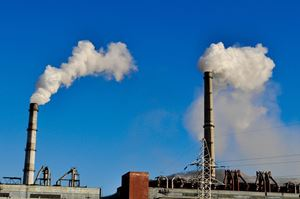Having examined how the price mechanism works in free market economies, we now turn our attention to the causes and consequences of market failure. This refers to a situation in which the market is unable to allocate resources efficiently. The actions of producers and consumers contribute to negative or positive effects on third parties.

#### 47 Practice Exercise 24 Solutions 05:13

This video lesson contains guidelines on how to respond to questions from the practice exercise for lesson 24.

#### 48 Lesson 25: Market Failure- Positive Externalities 29:22This lesson uses market diagrams to explain how the actions of producers and consumers affect third parties positively. For example, firms that train their workers may benefit other companies to which those workers move. Such companies also help to improve the quality of the country's pool of human resources.

#### 49 Practice Exercise 25 Solutions 04:13

This video lesson contains guidelines on how to respond to questions from the practice exercise for lesson 25.

#### 50 Lesson 26: Basic Private Sector Organisations 26:34This lesson- the first in unit 3- Business Economics- introduces the sub-topics that will be covered in the unit. These range from basic business organisations and how they compete to employment and wages as well as banking institutions. The lesson looks at how individuals start businesses and the questions they have to answer. it also explores the issues a business needs as well as its objectives before analysing the advantages and disadvantages of sole trader and partnership businesses.

#### 51 Practice Exercise 26 Solutions 04:22

This video lesson contains guidelines on how to respond to questions from the practice exercise for lesson 26.

#### 52 Lesson 27: Limited Companies 16:09Learning Objectives 1. To explain the meaning of incorporation 2. To distinguish between private and public limited companies 3. To analyse the advantages and disadvantages of limited companies

#### 53 Practice Exercise 27 Solutions 04:10

This video lesson contains guidelines on how to respond to questions from the practice exercise for lesson 27.

#### 54 Lesson 28: Multinational Corporations 25:21We now look at a type of business organisation whose role in global business continues to increase with the spread of globalisation. Multinational corporations (MNCs) are businesses with operations in more than one country. This lesson discusses the reasons a business would like to become a multinational as well as the benefits and limitations to both the busin

#### 55 Practice Exercise 28 Solutions 06:39

Solutions to Practice exercise 28

#### 56 Lesson 29: The Size and Growth of Firms 28:38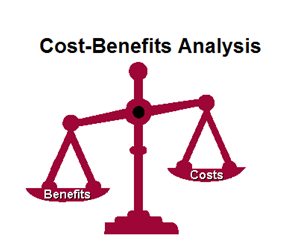We identify the different factors used to measure the size of firms. The lesson then examines the internal and external methods used by firms to expand in size before considering the benefits and costs of growth also known as economies and diseconomies of scale.

#### 57 Practice Exercise 29 Solutions 06:13

This video lesson contains guidelines on how to respond to questions from the practice exercise for lesson 29.

#### 58 Lesson 30: Production Costs in the Short and Long Run 29:40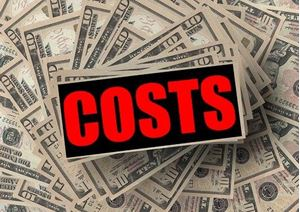The lesson starts with a distinction between the short and long run periods in production. It then considers the different types of costs incurred in production before examining the economies and diseconomies of scale

#### 59 Practice Exercise 30 Solutions 03:42

This video lesson contains guidelines on how to respond to questions from the practice exercise for lesson 30.

#### 60 Lesson 31: Revenues, Profits and Goals of Firms 26:09In this lesson, we seek to understand how businesses make revenues and what they spend these on. We also distinguish between the accountant's and economist's profits before looking at the different types of economic profits.

#### 61 Practice Exercise 31 Solutions 03:08

This video lesson contains guidelines on how to respond to questions from the practice exercise for lesson 31.

#### 62 Lesson 32: Perfect Competition and Monopoly 28:47We examine the features of two market structures- monopoly and oligopoly and compare their revenues and cost structures both in the short and long run.

#### 63 Practice Exercise 32 Solutions 05:42

This video lesson contains guidelines on how to respond to questions from the practice exercise for lesson 32.

#### 64 Lesson 33: Oligopoly and Monopolistic Competition 27:56In our examination of market structures, we explore the features of oligopolistic firms and compare them with those of a monopolistic competition structure. We end the lesson with a comprehensive comparison of the four market structures.

#### 65 Practice Exercise 33 Solutions 04:30

Solutions to Practice Exercise 33

#### 66 Lesson 34: Employment and Wages 25:52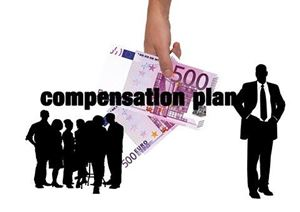This lessons turns our attention to employment and occupations. We look at the wage and non-wage factors that attract workers to a business organisation. We also consider measures taken by the government to correct market failure in the labour market.

#### 67 Practice Exercise 34 Solutions 04:27

This video lesson contains guidelines on how to respond to questions from the practice exercise for lesson 34.

#### 68 Lesson 35: Wage Differentials 26:05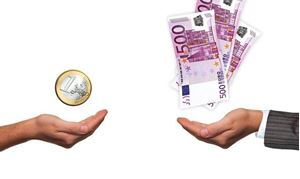Learning Outcomes 1. To define occupational wage differentials and explain what causes them. 2. To explain why earnings differ between people doing the same job. 3. To analyse why governments intervene in labour markets.

#### 69 Practice exercise 35 Solutions 05:48

This video lesson contains guidelines on how to respond to questions from the practice exercise for lesson 35.

#### 70 Lesson 36: Money and Finance 26:16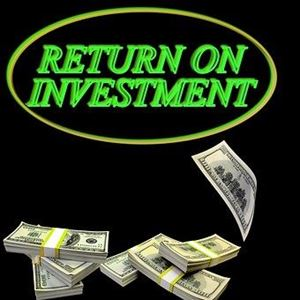In this lesson, we examine the functions of money and the role played by interest rates in attracting business investment.

#### 71 Practice Exercise 36 Solutions 03:22

This video lesson contains guidelines on how to respond to questions from the practice exercise for lesson 36.

#### 72 Lesson 37: Banking and the Financial Institutions 24:55This lesson defines financial institutions and the role they play in the money market. We also explain the role of commercial banks and how central banks control and regulate the financial system in an economy.

#### 73 Practice Exercise 37 Solutions 03:16

This video lesson contains guidelines on how to respond to questions from the practice exercise for lesson 37.

#### 74 Lesson 38: Consumer Behaviour 29:14LEARNING OBJECTIVES 1. To analyse the different motives for spending, saving and borrowing 2. To explain reasons for rising trends in global consumption

#### 75 Practice Exercise 38 Solutions 04:28

This video lesson contains guidelines on how to respond to questions from the practice exercise for lesson 38.

#### 76 Introduction to Section 4 03:25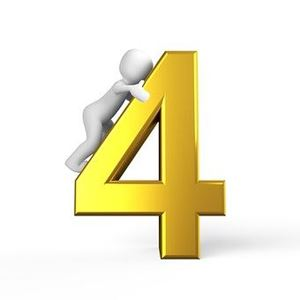This video provides a short introduction to the lessons to be covered in Unit 4- Macroeconomics

#### 77 Lesson 39: The Macroeconomy 27:55In this lesson, we revisit the circular flow of income and use it to examine economic concepts that impact upon the whole economy. The lesson looks at injections into and leakages from the circular flow of income. Using the circular flow model we then look at how economic activity is measured.

#### 78 Practice Exercise 39 Solutions 05:47

Solutions to Practice exercise 39

#### 79 Lesson 40: Measuring National Income 29:35

This lesson starts off with an exploration of the uses of national income statistics and its limitations. We then use diagrammatic analysis to describe the Aggregate Demand and its components. We then consider the reasons that cause the AD to shift (components of the AD).

#### 80 Practice Exercise 40 Solutions 04:55

Solutions to Practice Exercise 40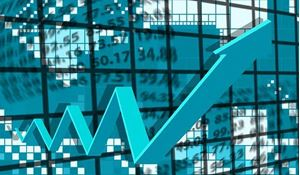In this lesson, we develop the concept of economic growth further using a formula to show how it is calculated. We then introduce the business cycle and consider the features of each of its phases before analysing its practical application.

#### 82 Practice Exercise 41 Solutions 05:23

Solutions to Practice exercise 41

#### 83 Lesson 42: Aggregate Supply 25:06

An illustration of the aggregate supply curve is used to explain the relationship between the price level and real output in the short-run. Shifts of the short-run aggregate supply curve and their effects are explored. The long run aggregate supply is also discussed with a distinction between the Keynesian and Neo classical schools of thought.

#### 84 Practice Exercise 42 Solutions 03:55

Solutions to Practice exercise 42

#### 85 Lesson 43: Macroeconomic Equilibrium 24:13In this lesson, we combine Aggregate Demand with Aggregate Supply to create the short-run and long-run macroeconomic equilibrium. In the short-run, we explore the impact of shifts in the AD and AS. The long-run analysis compares the Keynesian model to the Neo-classical/ monetarist model to explain equilibrium when a country reaches its potential output.

#### 86 Practice Exercise 43 Solutions 05:21

This video lesson contains guidelines on how to respond to questions in Practice exercise 43.

#### 87 Lesson 44: Macroeconomic Objectives and Economic Growth 22:41In this lesson, we introduce the five main economic objectives: high economic growth; low and stable prices; low unemployment; balance of payment and equitable distribution of income. We then embark on discussing GDP and economic growth.

#### 88 Practice Exercise 44 Solutions 04:22

This video lesson contains guidelines on how to respond to questions in Practice exercise 44.

#### 89 Lesson 45: Causes of and Measuring Unemployment 22:35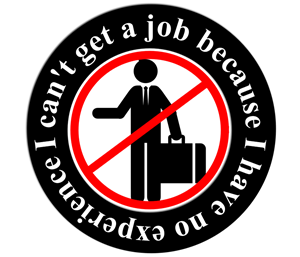We look at the second macroeconomic objective- low unemployment. We discuss key terminology relating to employment before outlining the methods used to calculate the rate of unemployment. We also discuss different types and causes of unemployment.

#### 90 Practice Exercise 45 Solutions 04:46

This video lesson contains guidelines on how to respond to questions in Practice exercise 45

#### 91 Lesson 46: Measures to Reduce Unemployment 27:54We look at the consequences of inflation on: a) the unemployed b) the government c) the economy. We also discuss different approaches to reducing the impact of unemployment.

#### 92 Practice Exercise 46 Solutions 03:19

This video contains a guideline on how to respond to the practice exercise for lesson 46.

#### 93 Lesson 47: The Consumer Price Index and Causes of Inflation 23:05We define inflation, deflation and disinflation before explaining how the consumer price index (CPI) is used to measure the rate of inflation. We also analyse the causes of inflation: demand pull and cost push inflation.

#### 94 Practice Exercise 47 Solutions 04:20

This video lesson contains guidelines on how to respond to questions in Practice exercise 47.

#### 95 Lesson 48: Consequences and Measures to Control Inflation 24:08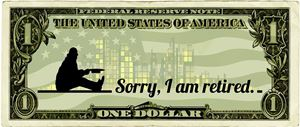This lesson examines the positive and negative consequences of inflation and deflation. It also analyses the effectiveness of measures used by the government to control inflation.

#### 96 Practice Exercise 48 Solutions 03:09

This video lesson contains guidelines on how to solve Practice exercise 48.

#### 97 Lesson 49: Equitable Distribution of Income 27:56In this lesson, we focus on the fourth macroeconomic objective- equitable distribution of income and wealth. We distinguish between equity and equality in the distribution of resources as well as identifying reasons for the inequality. We also examine the use of the Lorenz curve and Gini coefficient in measuring inequality.

#### 98 Practice Exercise 49 Solutions 04:07

Solutions to Practice exercise 49

#### 99 Lesson 50: Achieving Equitable Income Distribution 22:32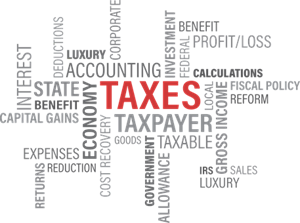Lesson Objectives 1. To distinguish between progressive, regressive and proportional tax systems 2. To explain how governments use tax revenues to redistribute income 3. To evaluate the effectiveness of measures used to promote equity in the distribution of income.

#### 100 Practice Exercise 50 Solutions 04:36

This video contains a guideline on how to respond to the practice exercise for lesson 50.

#### 101 Lesson 51: Fiscal Policy 25:17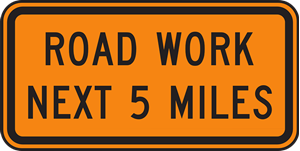Learning outcomes 1. To identify and explain sources of government revenue 2. To explain government spending and its role in achieving government objectives 3. To analyse the importance of government budgets.

#### 102 Practice Exercise 51 Solutions 04:06

This video contains a guideline on how to respond to the practice exercise for lesson 51.

#### 103 Lesson 52: Monetary Policy 18:15

Learning Outcomes 1. To define monetary policy and explain its tools 2. To distinguish between expansionary and contractionary fiscal policy 3. To assess the effectiveness of monetary policy tools

#### 104 Practice Exercise 52 Solutions 01:54

This video contains a guideline on how to respond to the practice exercise for lesson 52.

#### 105 Lesson 53: Supply-Side Policies 20:16Learning outcomes 1. To define and give examples of supply-side policies. 2. To explain how supply-side policies work to achieve macroeconomic objectives 3. To analyse conflicts that arise from application of macroeconomic policies

#### 106 Practice Exercise 53 Solutions 03:22

Solutions to Practice exercise 53

#### 107 Introduction to Section 5 04:19

This short lesson breaks down the contents of Section 5- International Trade and Economic Development.

#### 108 Lesson 54: The World Trade Organisation and Free Trade 13:32Learning Objectives: 1. To explain the meaning of international trade and specialisation 2. To explain the benefits that countries gain from trade 3. To explain the functions and objectives of the WTO.

#### 109 Practice Exercise 54 Solutions 05:07

This video lesson contains guidelines on how to respond to questions in Practice exercise 54.

#### 110 Lesson 55: Trade Protectionism 23:53Learning Objectives: 1. To define and give examples of trade protectionism 2. To explain the role of import tariffs in protecting trade 3. To explain non-tariff measures of protectionism

#### 111 Practice exercise 55 solutions 02:27

This video lesson contains guidelines on how to respond to questions in Practice exercise 55.

#### 112 Lesson 56: Free Trade Versus Protectionism 20:49Learning Objectives: 1. To discuss the arguments against trade protectionism 2. To discuss the arguments in favour of trade protectionism 3. To discuss the moral aspect in the argument in favour of free trade

#### 113 Practice Exercise 56 Solutions 03:24

This video lesson contains guidelines on how to respond to questions in Practice exercise 56.

#### 114 Lesson 57: Exchange Rates 17:54Learning Objectives: 1. To explain the meaning of exchange rates 2. To explain how value of an exchange rate is determined by demand and supply in a floating system 3. To discuss how governments intervene in the foreign exchange market

#### 115 Practice Exercise 57 Solutions 03:56

Solutions to Practice exercise 57

#### 116 Lesson 58: Economic Integration 27:54Learning Objectives: 1. To define and give examples of economic integration 2. To explain the stages of economic integration 3. To analyse the benefits and costs of economic integration

#### 117 Practice Exercise 58 Solutions 03:42

Solutions to Practice exercise 58.

#### 118 Lesson 59: The Global Population 29:22Learning Objectives: 1. To describe factors that affect population growth 2. To discuss the reasons for different rates of population growth in different countries 3. To analyse the consequences of these population changes for the different countries.

#### 119 Practice Exercise 59 Solutions 02:52

Solutions to Practice exercise 59.

#### 120 Lesson 60: Structure of the Population 19:10Learning Objectives 1. To describe the characteristics of populations 2. To explain the importance of population pyramids in explaining age and sex distribution 3. To illustrate population density and the occupational distribution of population.

#### 121 Practice Exercise 60 Solutions 03:14

Solutions to Practice exercise 60

#### 122 Lesson 61: Economic Development 13:30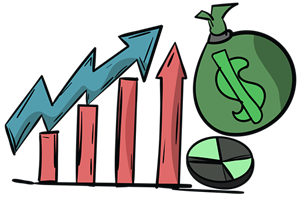Learning Objectives: 1. To distinguish between economic growth and development 2. To explain the multi-dimensional nature of economic development 3. To distinguish between economically more developed and less developed economies

#### 123 Practice Exercise 61 Solutions 02:21

Solutions to Practice exercise 61.

#### 124 Lesson 62: Common Characteristics of Developing Countries 24:05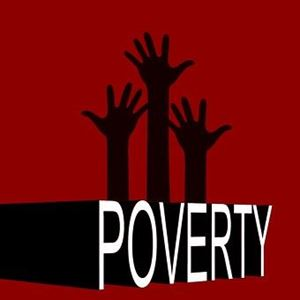Learning Objectives: 1. To explain, using examples, the common characteristics that less economically developed countries (LEDCs) share 2. To explain the meaning of the poverty trap- vicious cycle of poverty 3. To explain, using examples, that LEDCs differ enormously from each other.

#### 125 Practice Exercise 62 Solutions 03:00

Solutions to Practice exercise 62.

#### 126 Lesson 63: Measuring Economic Development 20:02Learning Objectives: 1. To explain the complexities of measuring economic development 2. To analyse the use of single indicators used as measures of economic development 3. To explain the measures that make up the Human Development Index (HDI)

#### 127 Practice Exercise 63 Solutions 04:47

Solutions to Practice Exercise 63

#### 128 Lesson 64: Domestic Barriers to Economic Development 17:34Learning Objectives: 1. To explain the role played by education and healthcare in the development of a nation 2. To analyse the need for appropriate technology and microcredit for economic development 3. To explain how poor infrastructure acts a barrier to economic development

#### 129 Practice Exercise 64 Solutions 05:12

Solutions to Practice exercise 64.

#### 130 Lesson 65: Trade Problems Facing LEDCs 12:34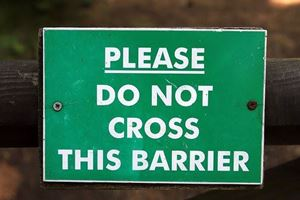Learning Objectives: 1. To explain how over-specialisation on a narrow range of products acts as an obstacle to economic development 2. To discuss the consequences of the inability of LEDCs to access international markets

#### 131 Practice Exercise 65 Solutions 05:17

This video lesson contains guidelines on how to respond to questions in Practice exercise 65.

#### 132 Lesson 66: Trade Strategies for Economic Development 20:22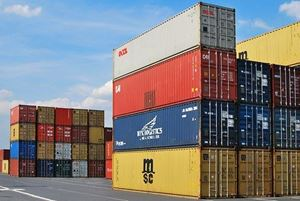Learning Objectives: 1. To evaluate the role of import substitution as a means to achieve economic development 2. To evaluate the role of export promotion as a means to achieve economic development 3. To evaluate the role of diversification as a means to achieve economic development

#### 133 Practice Exercise 66 Solutions 04:23

Solutions to Practice exercise 66.

#### 134 Lesson 67: Global Economic Challenges- Foreign Aid 20:15Learning Objectives: 1.To define the different types of foreign aid 2. To evaluate the contribution of foreign aid to economic development 3. To compare the merits of aid to those of trade for economic development

#### 135 Practice Exercise 67 Solutions 04:00

Solutions to Practice exercise 67

#### 136 Lesson 68: Global Economic Challenges- Foreign Debt 08:59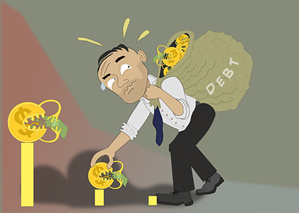Learning Objectives: 1. To explain the problem of foreign debt and its origins 2. To explain the consequences of a high level of debt 3. To evaluate the issue of debt cancellation in heavily indebted countries

#### 137 Practice Exercise 68 Solutions 04:20

Solutions to Practice exercise 68

#### 138 Lesson 69: Course Summary 11:12Learning Objectives: 1. To summarise the key concepts covered in each unit 2. To identify and explain important techniques to apply when completing the final examination

#### 139 Final Examination for Principles of Economics 00:00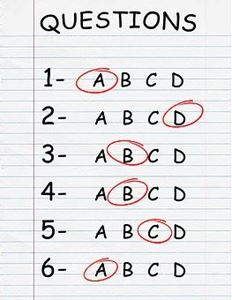This section contains the examination that YOU will complete at the end of the course. It is highly recommended that you complete the examination under timed examination conditions. Please ensure that you have no access to electronic gadgets (such as smart phones, tablets or i-watches). Also, you should not have access to any Economics text books. You are allowed 5 minutes at the beginning for you to read instructions and select the 2 questions that you are most comfortable completing. The five minutes are in addition to the 1 hour 30 minutes allocated for the examination.There are three questions but you only select two.

COURSE OVERVIEW

This "Principles of Economics" video course covers all of the contente students need to successfully gain a deep understanding of Economics at grade 10, 11 or 12. Students will gain critical thinking skills that will help them question economic events happening in their national economies as well as globally. The course will enrich their understanding of a broad array of economic concepts as well as prepare them for further studies in Economics at university.

This course contains:

•   68 VIDEO LESSONS (Over 25 hours of recorded lessons)
•   68 PRACTICE EXERCISES
•   68 PRACTICE EXERCISE SOLUTIONS (written)
•   68 PRACTICE EXERCISE EXPLANATIONS (video; I review each answer step by step and provide clarity where needed)
•   68 MULTIPLE CHOICE ONLINE QUIZZES
•   68 ANSWER KEYS TO THE MULTIPLE CHOCE QUESTIONS
•   OVER 30 Word, PDF & PPT SUMMARY NOTES
•   1 OVERALL COURSE SUMMARY
•   1 FINAL EXAM MARK SCHEME
•   1 FINAL EXAM VIDEO GUIDELINE

COURSE GOALS

Upon completion of this course, you wil gain lifelong skills including:

•        developing an understanding of basic economic concepts, terminology and principles
•        ability to understand their relevance to us as economic agents
•        ability to apply the tools of economic analysis
•        ability to distinguish between facts and value judgements in economic issues
•        ability to use mathematical and economic models to develop your understanding of real world issues
•        ability t to develop logical and coherent arguments when discussing economic issues.
•        ability to distinguish between the two main branches of Economics: Microeconomics and Macroeconomics
•        developing an understanding of microeconomic and macroeconomic concepts and theories and their real world application
•        developing an appreciation of the impact on individuals and societies of economic interactions between nations
•        developing empathy and sensitivity towards disadvantaged groups such as the poor and unemployed
•        developing an awareness of development issues facing nations as the undergo the process of change
•        ability to take a greater part in decision-making processes in every day life
•        developing a desire to study and understand human decisions and the nature of their relationships
•        acquiring an excellent foundation for advanced study in economics.

TARGET AUDIENCE

This video-course is intended for 11th and 12th grade students who would like to strengthen their conceptual understanding of Economics in preparation for university studies.

COURSE REQUIREMENTS

The first section of this course covers a wide variety of basic economic concepts and ideas, laying a solid foundation for the study of the other four sections. Students do not need to have covered an Economics course in advance of this course as it has been simplified to benefit beginners and those who might have covered an Economics course before.

UNIT 1: FOUNDATIONS OF ECONOMICS- The section contains key concepts and themes that will be revisited throughout the course as well as establishing the rationale for the classification of Economics as a social science.

1.  Introduction to Economics

2.  Microeconomics vs Macroeconomics

3.  Model-building in Economics

4.  Positive vs Normative Economics

5.  Basic Organizations & Economic Institutions

6.  The Basic Economic Problem

7.  Factor Markets: Factors of Production

8.  Product Markets: Types of Goods

9.  Opportunity Cost and The Production Possibilities Curve

10.  Key Themes in Economics & Why Economists Disagree

11.  What Questions Do Economic Systems Seek to Answer?

12.  Economic Systems & Decision-Making

13.  Free Market Economic System

14.  Planned & Mixed Economic Systems

UNIT 2: MICROECONOMICS- deals with decisions made by individual economic agents such as consumers (buyers) and producers (sellers).

15.  Competitive Markets & Demand

16.  Movements Along the Demand Curve

17.  The Determinants of Demand

18.  Elasticity of Demand

19.  The Supply Curve & Determinants of Supply

20.  Price Elasticity of Supply (PES)

21.  Price-Reaching an Equilibrium

22.  Linear Demand & Supply Functions

23.  Efficiency in the Market

24.  Market Failure: Negative Externalities

25.  Market Failure: Positive Externalities

UNIT 3: BUSINESS ECONOMICS- builds up on the concepts introduced in unit 2 by examining the different types of business organisations, how they set and achieve their objectives and how they compete with each other.

26.  Basic Private Sector Organizations

27.  Limited Companies

28.  Multinational Corporations

29.  The Size and Growth of Firms

30.  Production Costs in the Short & Long Run

31.  Revenues, Profits & Goal of Firms

32.  Market Structures: Perfect Competition & Monopoly

33.  Market Structures: Oligopoly & Monopolistic Competition

34.  Employment & Wages

35.  Wage Differentials & Government Intervention in the Labour Market

36.  Money & Finance

37.  Banking & The Financial Institutions

38.  Consumer Behaviour

UNIT 4: MACROECONOMICS- examines the second branch of the subject which concerns itself with decisions involving larger sections of the national and international economy.

39.  The Macro-economy & Government Objectives

40.  Measuring National Income: Aggregate Demand

42.  Aggregate Supply

43.  Macroeconomic Equilibrium

44.  Macroeconomic Objectives & Economic Growth

45.  Unemployment: Measuring & Causes of Unemployment

46.  Unemployment: Consequences of & Measure to Reduce Unemployment

47.  Inflation: The Consumer Price Index & Causes of Inflation

48.  Inflation: The consequences of & Measures Used to Control Inflation

49.  Equitable Distribution of Income: Meaning & Measurement

50.  Equitable Distribution of Income: Impact & Policies to Achieve Equity

51.  Macroeconomic Policies: Fiscal Policy

52.  Macroeconomic Policies: Monetary Policy

53.  Macroeconomic Policies: Supply-Side Policies

UNIT 5: INTERNATIONAL TRADE & DEVELOPMENT ECONOMICS- combines concepts from the previous four sections to discuss global economic challenges such as the need to protect trade from imports, economic development (and the lack of it) as well as strategies used to achieve economic development in LEDCs.

57.  Exchange rates

58.  Economic Integration

59.  The Global Population

60.  Structure of the Population

61.  Economic Development

62.  Common Characteristics of Developing Countries

63.  Measuring Economic Development

64.  Role of Domestic Factors in Economic Development

66.  Trade Strategies for Economic Growth & Development

67.  Global Economic Challenges – Foreign Aid

68.  Global Economic Challenges – Foreign Debt

69.  Course Summary

70.  Principles of Economics Final Examination

• Teacher: JOSEPH
• Areas of expertise: I have been teaching British A Levels and the International Baccalaureate (Economics and Business Management) for a total of 18 years now.
• Education: 1. Masters in Business Administration (MBA) from Tongji University, Shanghai- China; 2. Post Graduate Certificate in Education (PGCE) from University of Sunderland UK; 3. Bachelor of Arts (Economics) from Kenyatta University, Kenya.
• Interests: Soccer, reading, board games and spending time with family.
• Skills:
• Associations:
• Issues I care about: To create a positive impact on the students that I interact with by making them to love learning and prepare them to face the challenges of an ever-changing world.

In my 18 years of teaching in international schools in Kenya and China, I have interacted with students who have a passion to succeed in various spheres of life and a desire to be the best they can be. I have taught Business Management, Economics and Theory of Knowledge and always cherish the opportunity to see knowledge acquired being shared and applied to solve real world problems.

#### Practice Exercise Solutions 1

This document contains suggested answers to the practice exercise 1. Suggestions on how students gain marks for each question are given as well.

#### Practice Exercise Solutions 2

This document contains suggested answers to the practice exercise 2. Suggestions on how students gain marks for each question are given as well.

#### Practice Exercise Solutions 3

This document contains suggested answers to the practice exercise 3. Suggestions on how students gain marks for each question are given as well.

#### Practice Exercise Solutions 4

This document contains suggested answers to the practice exercise 4. Suggestions on how students gain marks for each question are given as well

#### Practice Exercise Solutions 5

This document contains suggested answers to the practice exercise 5. Suggestions on how students gain marks for each question are given as well

#### Practice Exercise Solutions 6

This document contains suggested answers to the practice exercise 6. Suggestions on how students gain marks for each question are given as well

#### Practice Exercise Solutions 7

This document contains suggested answers to the practice exercise 7. Suggestions on how students gain marks for each question are given as well.

#### Practice Exercise Solutions 8

This document contains suggested answers to the practice exercise 8. Suggestions on how students gain marks for each question are given as well.

#### Practice Exercise Solutions 9

This document contains suggested answers to the practice exercise 9. Suggestions on how students gain marks for each question are given as well.

#### Practice Exercise Solutions 10

This document contains suggested answers to the practice exercise 10. Suggestions on how students gain marks for each question are given as well.

#### Practice exercises Solutions 11

This document contains suggested answers to the practice exercise 11. Suggestions on how students gain marks for each question are given as well.

#### Practice Exercise Solutions 12

This document contains suggested answers to the practice exercise 12. Suggestions on how students gain marks for each question are given as well.

#### Practice Exercise Solutions 13

This document contains suggested answers to the practice exercise 13. Suggestions on how students gain marks for each question are given as well.

#### Practice Exercise Solutions 14

This document contains suggested answers to the practice exercise 14. Suggestions on how students gain marks for each question are given as well.

#### Practice Exercise Solutions 15

This document contains suggested answers to the practice exercise 15. Suggestions on how students gain marks for each question are given as well.

#### Practice Exercise Solutions 16

This document contains suggested answers to the practice exercise 16. Suggestions on how students gain marks for each question are given as well.

#### Practice Exercise Solutions 17

This document contains suggested answers to the practice exercise 17. Suggestions on how students gain marks for each question are given as well.

#### Practice Exercise Solutions 18

This document contains suggested answers to the practice exercise 18. Suggestions on how students gain marks for each question are given as well.

#### Practice Exercise Solutions 19

This document contains suggested answers to the practice exercise 19. Suggestions on how students gain marks for each question are given as well.

#### Practice Exercise Solutions 20

This document contains suggested answers to the practice exercise 20. Suggestions on how students gain marks for each question are given as well.

#### Practice Exercise Solutions 21

This document contains suggested answers to the practice exercise 21. Suggestions on how students gain marks for each question are given as well.

#### Practice Exercise Solutions 22

This document contains suggested answers to the practice exercise 22. Suggestions on how students gain marks for each question are given as well.

#### Practice Exercise Solutions 23

This document contains suggested answers to the practice exercise 23. Suggestions on how students gain marks for each question are given as well.

#### Practice Exercise Solutions 24

This document contains suggested answers to the practice exercise 24. Suggestions on how students gain marks for each question are given as well.

#### Practice Exercise Solutions 25

This document contains suggested answers to the practice exercise 25. Suggestions on how students gain marks for each question are given as well.

#### Practice Exercise Solutions 26

This document contains suggested answers to the practice exercise 26. Suggestions on how students gain marks for each question are given as well.

#### Practice Exercise Solutions 27

This document contains suggested answers to the practice exercise 27. Suggestions on how students gain marks for each question are given as well.

#### Practice Exercise Solutions 28

This document contains suggested answers to the practice exercise 28. Suggestions on how students gain marks for each question are given as well.

#### Practice Exercise Solutions 29

This document contains suggested answers to the practice exercise 29. Suggestions on how students gain marks for each question are given as well.

#### Practice Exercise Solutions 30

This document contains suggested answers to the practice exercise 30. Suggestions on how students gain marks for each question are given as well.

#### Practice Exercise Solutions 31

This document contains suggested answers to the practice exercise 31. Suggestions on how students gain marks for each question are given as well.

#### Practice Exercise Solutions 32

This document contains suggested answers to the practice exercise 32. Suggestions on how students gain marks for each question are given as well.

#### Practice Exercise Solutions 33

This document contains suggested answers to the practice exercise 33. Suggestions on how students gain marks for each question are given as well.

#### Practice Exercise Solutions 34

This document contains suggested answers to the practice exercise 34. Suggestions on how students gain marks for each question are given as well.

#### Practice Exercise Solutions 35

This document contains suggested answers to the practice exercise 35. Suggestions on how students gain marks for each question are given as well.

#### Practice Exercise Solutions 36

This document contains suggested answers to the practice exercise 36. Suggestions on how students gain marks for each question are given as well.

#### Practice Exercise Solutions 37

This document contains suggested answers to the practice exercise 37. Suggestions on how students gain marks for each question are given as well.

#### Practice Exercise Solutions 38

This document contains suggested answers to the practice exercise 38. Suggestions on how students gain marks for each question are given as well.

#### Practice Exercise Solutions 39

This document contains suggested answers to the practice exercise 39. Suggestions on how students gain marks for each question are given as well.

#### Practice Exercise Solutions 40

This document contains suggested answers to the practice exercise 40. Suggestions on how students gain marks for each question are given as well.

#### Practice Exercise Solutions 41

This document contains suggested answers to the practice exercise 41. Suggestions on how students gain marks for each question are given as well.

#### Practice Exercise Solutions 42

This document contains suggested answers to the practice exercise 42. Suggestions on how students gain marks for each question are given as well.

#### Practice Exercise Solutions 43

This document contains suggested answers to the practice exercise 43. Suggestions on how students gain marks for each question are given as well.

#### Practice Exercise Solutions 44

This document contains suggested answers to the practice exercise 44. Suggestions on how students gain marks for each question are given as well.

#### Practice Exercise Solutions 45

This document contains suggested answers to the practice exercise 45. Suggestions on how students gain marks for each question are given as well.

#### Practice Exercise Solutions 46

This document contains suggested answers to the practice exercise 46. Suggestions on how students gain marks for each question are given as well.

#### Practice Exercise Solutions 47

This document contains suggested answers to the practice exercise 47. Suggestions on how students gain marks for each question are given as well.

#### Practice Exercise solutions 48

This document contains suggested answers to the practice exercise 48. Suggestions on how students gain marks for each question are given as well.

#### Practice Exercise Solutions 49

This document contains suggested answers to the practice exercise 49. Suggestions on how students gain marks for each question are given as well.

#### Practice Exercise Solutions 50

This document contains suggested answers to the practice exercise 50. Suggestions on how students gain marks for each question are given as well.

#### Practice Exercise Solutions 51

This document contains suggested answers to the practice exercise 51. Suggestions on how students gain marks for each question are given as well.

#### Practice Exercise Solutions 52

This document contains suggested answers to the practice exercise 52. Suggestions on how students gain marks for each question are given as well.

#### Practice Exercise Solutions 53

This document contains suggested answers to the practice exercise 53. Suggestions on how students gain marks for each question are given as well.

#### Practice Exercise Solutions 54

This document contains suggested answers to the practice exercise 54. Suggestions on how students gain marks for each question are given as well.

#### Practice Exercise Solutions 55

This document contains suggested answers to the practice exercise 55. Suggestions on how students gain marks for each question are given as well.

#### Practice Exercise Solutions 56

This document contains suggested answers to the practice exercise 56. Suggestions on how students gain marks for each question are given as well.

#### Practice Exercise Solutions 57

This document contains suggested answers to the practice exercise 57. Suggestions on how students gain marks for each question are given as well.

#### Practice Exercise Solutions 58

This document contains suggested answers to the practice exercise 58. Suggestions on how students gain marks for each question are given as well.

#### Practice Exercise Solutions 59

This document contains suggested answers to the practice exercise 59. Suggestions on how students gain marks for each question are given as well.

#### Practice Exercise Solutions 60

This document contains suggested answers to the practice exercise 60. Suggestions on how students gain marks for each question are given as well.

#### Practice Exercise Solutions 61

This document contains suggested answers to the practice exercise 61. Suggestions on how students gain marks for each question are given as well.

#### Practice Exercise Solutions 62

This document contains suggested answers to the practice exercise 62. Suggestions on how students gain marks for each question are given as well.

#### Practice Exercise Solutions 63

This document contains suggested answers to the practice exercise 63. Suggestions on how students gain marks for each question are given as well.

#### Practice Exercise Solutions 64

This document contains suggested answers to the practice exercise 64. Suggestions on how students gain marks for each question are given as well.

#### Practice Exercise Solutions 65

This document contains suggested answers to the practice exercise 65. Suggestions on how students gain marks for each question are given as well.

#### Practice Exercise Solutions 66

This document contains suggested answers to the practice exercise 66. Suggestions on how students gain marks for each question are given as well.

#### Practice Exercise Solutions 67

This document contains suggested answers to the practice exercise 67. Suggestions on how students gain marks for each question are given as well.

#### Practice Exercise Solutions 68

This document contains suggested answers to the practice exercise 68. Suggestions on how students gain marks for each question are given as well.

#### PRINCIPLES OF ECONOMICS EXAMINATION

This section contains the examination that students will complete at the end of the course. Students are expected to complete the examination under timed examination conditions. Please ensure that they have no access to electronic gadgets (such as smart phones, tablets or iwatches). Also, they should not have access to any Economics text books. Allow them 5 minutes at the beginning for them to read instructions and select the 2 questions they are most comfortable completing. The mark scheme for this examination is also attached.

#### Course examination assessment guide

This video explains how to use the Course examination mark scheme to assess the final examination.

#### Test 68 Key